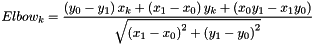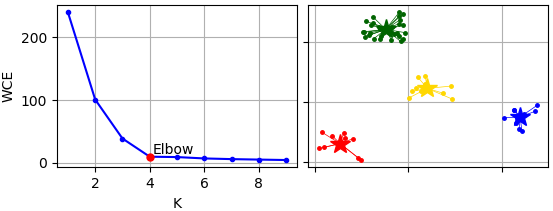pyclustering.cluster.elbow.elbow Class Reference

Class represents Elbow method that is used to find out appropriate amount of clusters in a dataset. More...

## Public Member Functions

def __init__ (self, data, kmin, kmax, kwargs)
Construct Elbow method. More...

def process (self)
Performs analysis to find out appropriate amount of clusters. More...

def get_amount (self)
Returns appropriate amount of clusters.

def get_wce (self)
Returns list of total within cluster errors for each K-value (kmin, kmin + 1, ..., kmax - 1).

## Detailed Description

Class represents Elbow method that is used to find out appropriate amount of clusters in a dataset.

Elbow method performs clustering using K-Means algorithm for each K and estimate clustering results using sum of square erros. By default K-Means++ algorithm is used to calculate initial centers that are used by K-Means algorithm.

The Elbow is determined by max distance from each point (x, y) to segment from kmin-point (x0, y0) to kmax-point (x1, y1), where 'x' is K (amount of clusters), and 'y' is within-cluster error. Following expression is used to calculate Elbow length:Usage example of Elbow method for cluster analysis:

from pyclustering.cluster.kmeans import kmeans, kmeans_visualizer
from pyclustering.cluster.center_initializer import kmeans_plusplus_initializer
from pyclustering.cluster.elbow import elbow
from pyclustering.samples.definitions import SIMPLE_SAMPLES
# read sample 'Simple3' from file (sample contains four clusters)
# create instance of Elbow method using K value from 1 to 10.
kmin, kmax = 1, 10
elbow_instance = elbow(sample, kmin, kmax)
# process input data and obtain results of analysis
elbow_instance.process()
amount_clusters = elbow_instance.get_amount() # most probable amount of clusters
wce = elbow_instance.get_wce() # total within-cluster errors for each K
# perform cluster analysis using K-Means algorithm
centers = kmeans_plusplus_initializer(sample, amount_clusters,
amount_candidates=kmeans_plusplus_initializer.FARTHEST_CENTER_CANDIDATE).initialize()
kmeans_instance = kmeans(sample, centers)
kmeans_instance.process()
# obtain clustering results and visualize them
clusters = kmeans_instance.get_clusters()
centers = kmeans_instance.get_centers()
kmeans_visualizer.show_clusters(sample, clusters, centers)

By default Elbow uses K-Means++ initializer to calculate initial centers for K-Means algorithm, it can be changed using argument 'initializer':

# perform analysis using Elbow method with random center initializer for K-Means algorithm inside of the method.
kmin, kmax = 1, 10
elbow_instance = elbow(sample, kmin, kmax, initializer=random_center_initializer)
elbow_instance.process()Elbows analysis with further K-Means clustering.

Definition at line 37 of file elbow.py.

## ◆ __init__()

 def pyclustering.cluster.elbow.elbow.__init__ ( self, data, kmin, kmax, kwargs )

Construct Elbow method.

Parameters
 [in] data (array_like): Input data that is presented as array of points (objects), each point should be represented by array_like data structure. [in] kmin (int): Minimum amount of clusters that should be considered. [in] kmax (int): Maximum amount of clusters that should be considered. [in] **kwargs Arbitrary keyword arguments (available arguments: 'ccore', 'initializer').

Keyword Args:

• ccore (bool): If True then CCORE (C++ implementation of pyclustering library) is used (by default True).
• initializer (callable): Center initializer that is used by K-Means algorithm (by default K-Means++).

Definition at line 94 of file elbow.py.

## ◆ process()

 def pyclustering.cluster.elbow.elbow.process ( self )

Performs analysis to find out appropriate amount of clusters.

Returns

Definition at line 133 of file elbow.py.

The documentation for this class was generated from the following file: